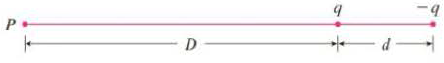Chapter 11.11, Problem 33E

Chapter
Section
Textbook Problem

# An electric dipole consists of two electric charges of equal magnitude and opposite sign. If the charges are q and −q and are located at a distance d from each other, then the electric field E at the point P in the figure is E = q D 2 − q ( D + d ) 2 By expanding this expression for E as a series in powers of d/D, show that E is approximately proportional to 1/D3 when P is far away from the dipole.To determine

To show: The Electric Field E is approximately proportional to 1D3, when P is far from the dipole.

Explanation

Given that the electric field E at point P is E=qD2q(D+d)2.

Expand E=qD2q(D+d)2 as follows.

E=qD2q(D+d)2=qD2qD2(1+dD)2=qD2(1(1+dD)2)

Use Binomial series and expand (1+dD)2 as follows.

(1+dD)2=(12(dD)+2×32!(dD)22×3×43!(dD)3+)

The value of D is large when compared with d

### Still sussing out bartleby?

Check out a sample textbook solution.

See a sample solution

#### The Solution to Your Study Problems

Bartleby provides explanations to thousands of textbook problems written by our experts, many with advanced degrees!

Get Started

#### solve the equation by using the quadratic formula. 135. 4x2 + 5x 6 = 0

Applied Calculus for the Managerial, Life, and Social Sciences: A Brief Approach

#### Evaluate the integral. 25. 4xx3+x2+x+1dx

Single Variable Calculus: Early Transcendentals

#### The area of the region bounded by , , and r = sec θ is:

Study Guide for Stewart's Multivariable Calculus, 8th